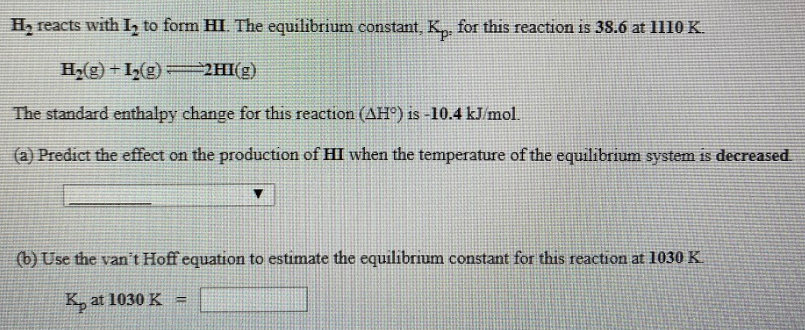# H2 reacts with I2 to form HI. The equilibrium constant, Kp, for this reaction is 38.6 at 1110 K. H2(g) + I2(g) ⇌ 2HI(g) The standard enthalpy change for this reaction (ΔH°) is -10.4 kJ/mol. (a) Predict the effect on the production of HI when the temperature of the equilibrium system decreased. (b) Use the van't Hoff equation to estimate the equilibrium constant for this reaction at 1030 K.